Year 9 Interactive Maths - Second Edition

## Similar Triangles

If the angles of one triangle are equal to the angles of another triangle, then the triangles are said to be equiangular.

Equiangular triangles have the same shape but may have different sizes.  So, equiangular triangles are also called similar triangles.

For example, triangle DEF is similar to triangle ABC as their three angles are equal.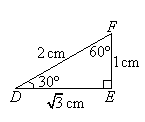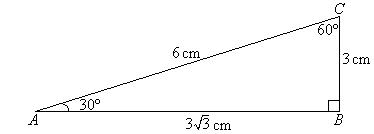The length of each side in triangle DEF is multiplied by the same number, 3, to give the sides of triangle ABC.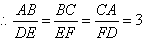###### In general:

If two triangles are similar, then the corresponding sides are in the same ratio.

#### Example 26

Find the value of x in the following pair of triangles.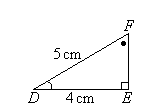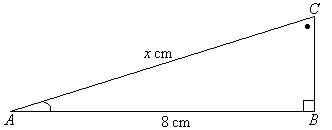##### Solution: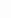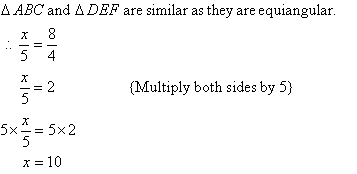###### Note:

Equal angles are marked in the same way in diagrams.

#### Example 27

Find the value of the pronumeral in the following diagram.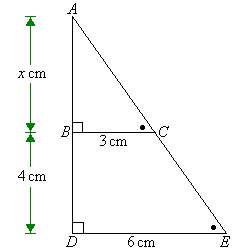##### Solution: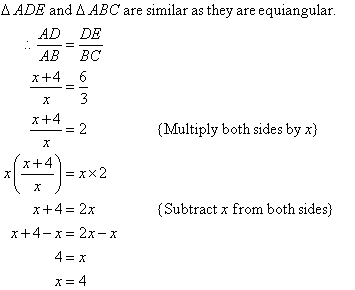### Applications of Similarity

Similar triangles can be applied to solve real world problems.

#### Example 28

Find the value of the height, h m, in the following diagram at which the tennis ball must be hit so that it will just pass over the net and land 6 metres away from the base of the net.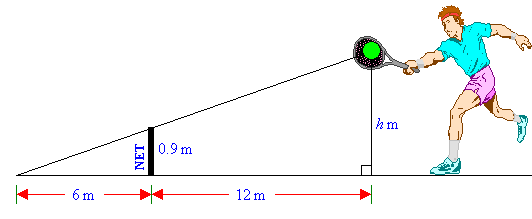##### Solution: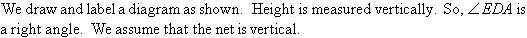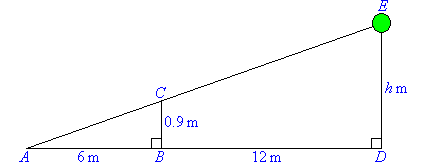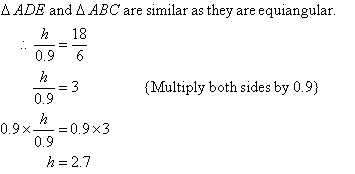So, the height at which the ball should be hit is 2.7 m.

###### Note:

a.  Equal angles are marked in the same way in diagrams.

b.  Two triangles are similar if:

• two pairs of corresponding sides are in the same ratio and the angle included between them are equal.

• the corresponding sides are in the same ratio.

• the corresponding angles are the same.